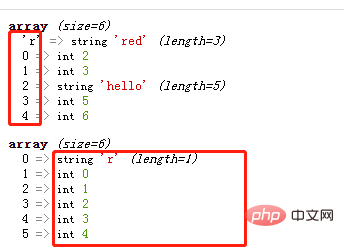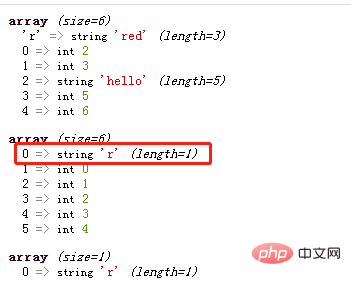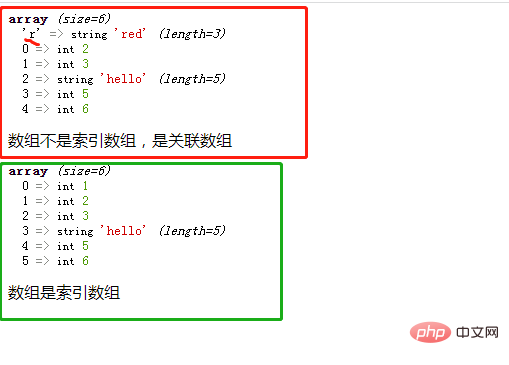# php怎么判断一个数组是否为索引数组php入门到就业线上直播课：进入学习

array_keys() 函数返回包含数组中所有键名的一个新数组。

`array_keys(array,value,strict)`

array必需。规定数组。
value可选。您可以指定键值，然后只有该键值对应的键名会被返回。
strict可选。与 value 参数一起使用。可能的值：
• true - 返回带有指定键值的键名。依赖类型，数字 5 与字符串 "5" 是不同的。
• false - 默认值。不依赖类型，数字 5 与字符串 "5" 是相同的。
```<?php
\$arr=array("r"=>"red",2,3,"hello",5,6);
var_dump(\$arr);
\$keys=array_keys(\$arr);
var_dump(\$keys);
?>``````function f(\$v){
return(is_string(\$v));
}
\$res=array_filter(\$keys,"f");
var_dump(\$res);````\$res==[]`
• 如果为空，则数组是索引数组

• 如果不为空，则数组不是索引数组，是关联数组

```<?php
function f(\$v){
return(is_string(\$v));
}
function fun(\$arr){
\$keys=array_keys(\$arr);

\$res=array_filter(\$keys,"f");
if(\$res==[]){
echo "数组是索引数组<br>";
}else{
echo "数组不是索引数组，是关联数组<br>";
}
}

\$arr=array("r"=>"red",2,3,"hello",5,6);
var_dump(\$arr);
fun(\$arr);
\$arr=array(1,2,3,"hello",5,6);
var_dump(\$arr);
fun(\$arr);
?>```1/1